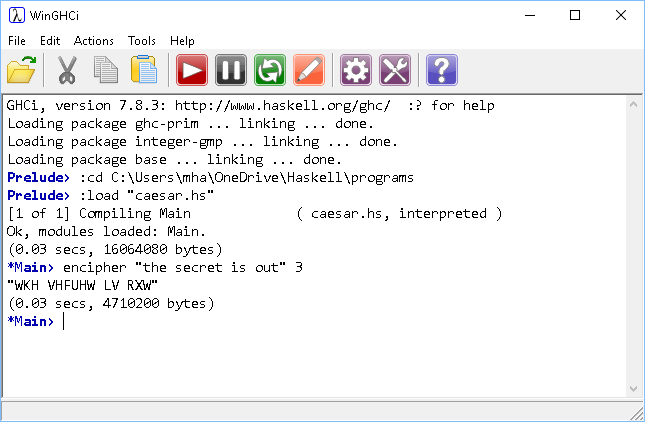# Introduction To Haskell Caesar Shift Cipher

## Introduction

Programming ciphering and deciphering algorithms is a good way to practise manipulating text with a programming language.

The Caesar Shift cipher is a simple substituion cipher. It gets its name from the way that the cipher alphabet is formed. It is a mono-alphabetic substitution cipher since each letter is replaced by only one other letter and the same one each time it appears in the unencrypted message.

The table shows a plain alphabet on the top row. The alphabet on the bottom row has been shifted 3 places to the right.

 Plain A B C D E F G H I J K L M N O P Q R S T U V W X Y Z Cipher D E F G H I J K L M N O P Q R S T U V W X Y Z A B C

To encrypt a message, replace every letter in the message with the one shown below it in the table. For example,

```THE SECRET IS OUT WKH VHFUHW LV RXW```

Using a Caesar Shift of +3, each letter of the plain text message is replaced with the letter 3 places to the right in the alphabet.

## Program

This one needs to import a package. This gives access to some functions for working with character data.

The ord function gives you the position of an item in whichever ordered structure it belonged to. With characters, you get the ASCII number.

The chr function returns a character when fed an ASCII number.

```import Data.Char

uppercase m = words (map (toUpper) m)

shiftedchar c n = chr((mod ((ord c - ord 'A') + n) 26) + ord 'A')
shiftedword w n = map (`shiftedchar` n) w

encipher m n = unwords(map (`shiftedword` n) (uppercase m))
```

In action,